# a new method for interpolation analysis of

## enzyme-linked immunosorbent assay (ELISA) data.

Abstract

We introduce a new method for the analysis of enzyme-linked immunosorbent assay (ELISA) data. The new method can use data near the asymptotes and does not give undue weight to responses on the flatter parts of the dose-response curve. We apply it to simulated data and to two real-world assays and show it is more accurate and more precise than the traditional interpolation method. In particular, the new method works much better for very low-concentration samples for which the traditional method is often unable to give a result.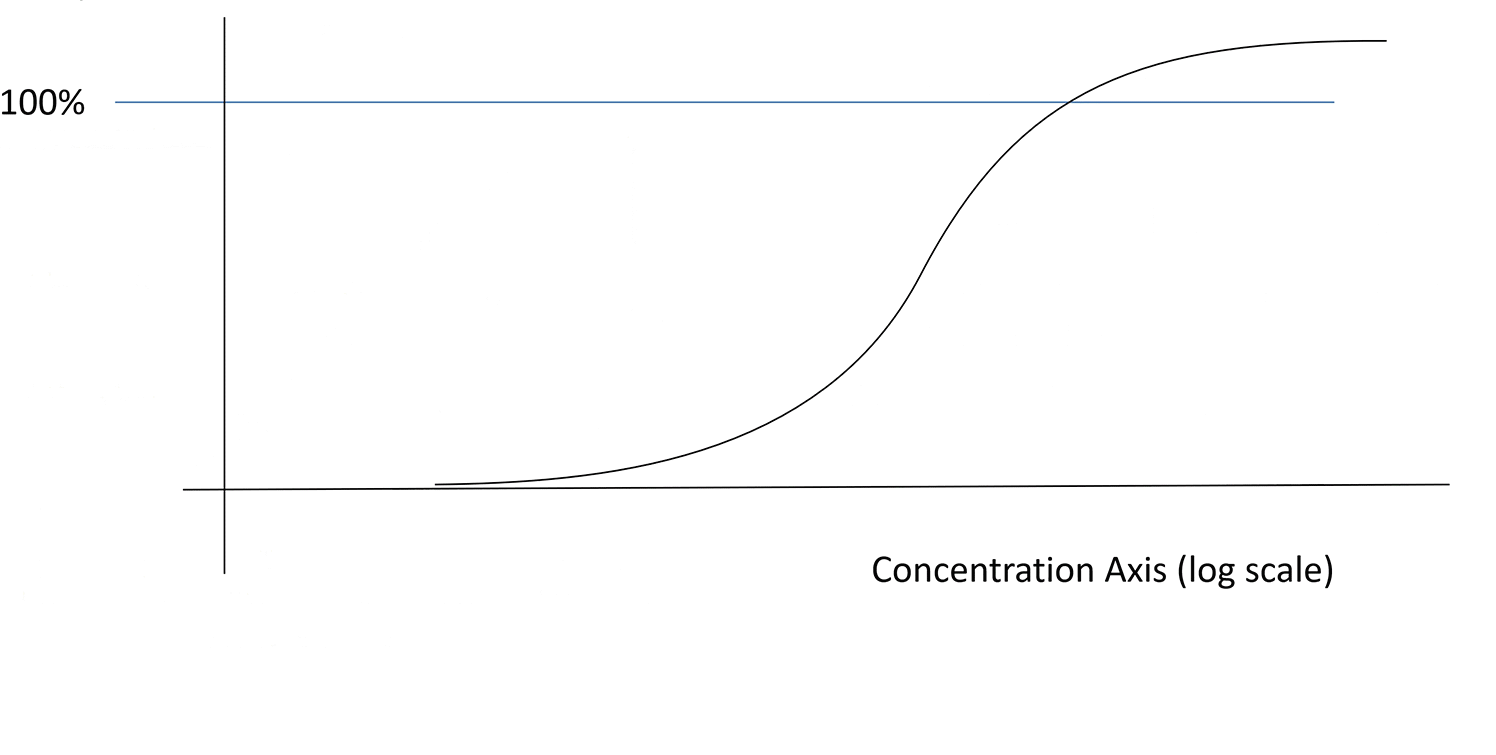1. Introduction

Enzyme-linked immunosorbent assays (ELISAs) are commonly used to quantify the amount of an unknown biological substance in a sample. ELISAs are often highly variable, so a direct analysis of the test sample responses would give very variable results. Instead, the analysis is carried out indirectly by comparing the responses for the test sample(s) to those of a reference standard. Traditionally, this is done using interpolation; a dose-response curve is fitted to the reference standard data and then this curve is used to interpolate the concentration of each dilution of the test sample independently (see Methods and materials section for details).

In most cases the interpolation method works well and gives accurate and precise results. However, there is still room for improvement. Making optimal use of the data can reduce the number of replicates and/or dilutions needed to achieve a given accuracy and precision. In this paper we present an alternative method which can achieve this. In brief, we fit a dose-response model to all the test sample data simultaneously, and extract the unknown test sample concentration from the parameters of the fit – the full details are presented in the Methods and materials section below. In addition, the interpolation method often uses complicated suitability criteria, and can fail to give a result (particularly for very low or high concentration samples). Our new method offers an opportunity to greatly simplify the suitability criteria, and is more likely to give a result over a wider range of concentrations.

To confirm that our new method indeed has superior accuracy and precision, we have applied both the traditional method and our newly proposed method to simulated data and real-world data. We compare the performance of the two methods in terms of accuracy, precision, and the proportion of samples passing suitability tests. The details of the simulated and real-worlds datasets are presented in the Methods and Materials section; the performance of the two methods is analysed in the Results section.

2. Methods and materials

The aim of both the traditional and new approaches is to calculate the unknown concentration U of a test sample. Typically an ELISA uses one or more dilutions di of the test sample, each of which may have one or more replicates. For each dilution and replicate there will be a response yi. The aim of the analysis is to estimate U, given the di and yi and the data for the reference standard.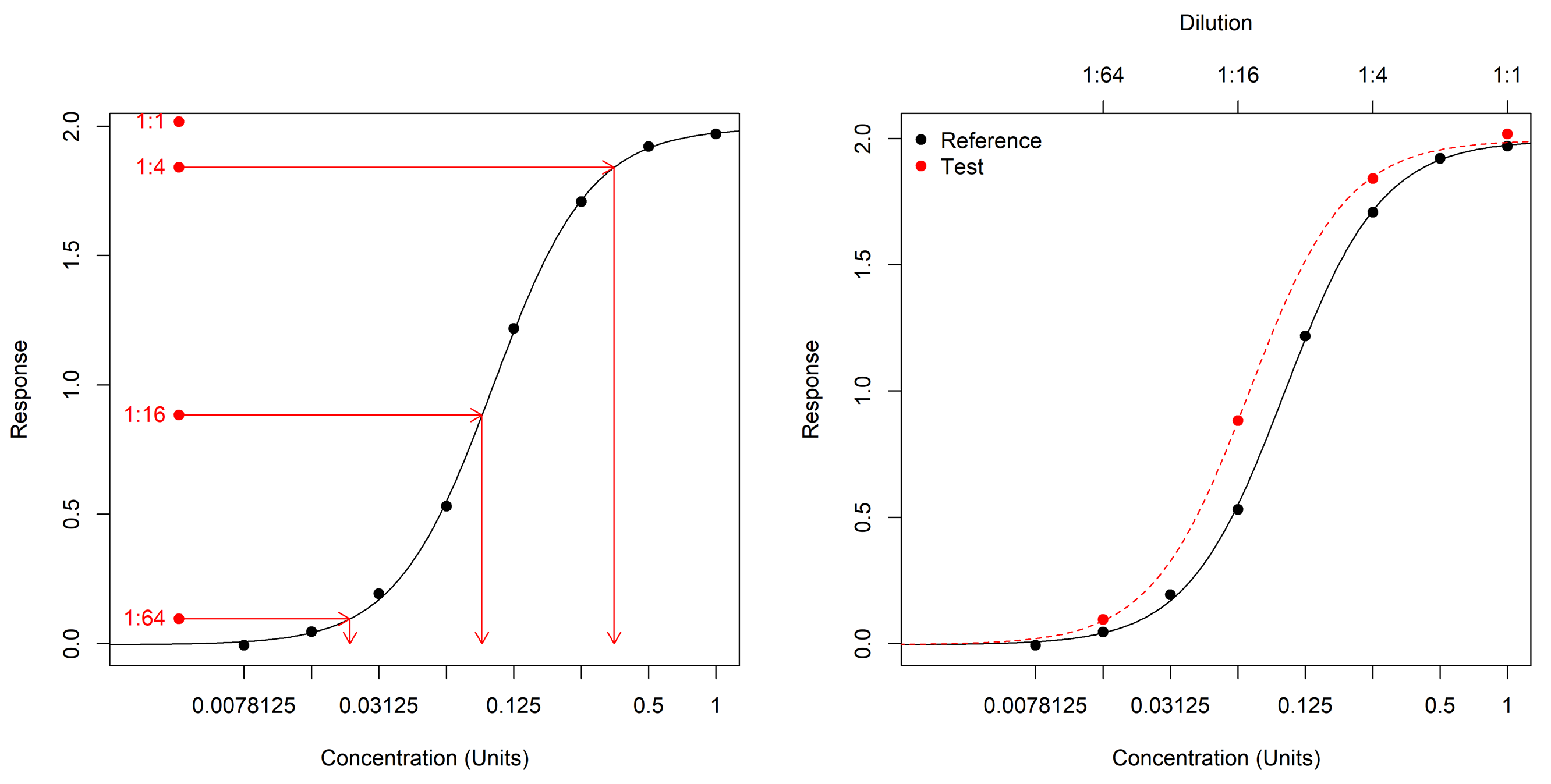Fig. 1. Example simulated assay, analysed using both the traditional and the new methods. Left panel: traditional method. Each test response (red points) is interpolated using the reference standard curve (black) to give a concentration estimate (arrows at bottom). Note the uppermost test response is above the upper reference asymptote so cannot be interpolated. Right panel: new method. The test fit (red curve) is a 4PL fit with the A, B and D parameters fixed to their values from the reference standard model (black curve).

2.1. Traditional (interpolation) approach to ELISA analysis

The traditional approach proceeds as follows. First a dose-response model is fitted to the reference standard. Usually either a linear or a four-parameter logistic (4PL) model is used, though other models are also possible. In this paper we will consider only the 4PL, but everything we will say applies to other methods as well.

The 4PL model for the reference standard is:

$$\text {response}=D+\frac{A-D}{1+\exp \left(B\left(\ln (\text { concentration })-C_{\mathrm{ref}}\right)\right)}$$

Here A is the left asymptote, D is the right asymptote, B is the slope parameter, and Cref is the midpoint (EC50) of the curve. The best fitting parameters A, B, Cref and D are found by fitting all the reference data simultaneously to this model, usually using statistical software.

The model is then used to estimate concentrations for each test sample. If a test sample has multiple dilutions and/or replicates, each of these is treated separately. The concentration of the test sample is estimated by interpolation. That is, the concentration estimate is the point on the reference standard curve which gives the observed response. This is illustrated in Fig. 1.

In the case of the 4PL, the interpolated concentration, xi, corresponding to a test response yi is given by

$$\ln \left(x_{i}\right)=C_{r e f}+\frac{1}{B} \ln \left(\frac{A-y_{i}}{y_{i}-D}\right)$$

To estimate the original test sample concentration, xi is then multiplied by the test sample dilution di. For example, in the left panel of Fig. 1, xi for the first point is 0.0232; this is multiplied by 64 to give a concentration estimate of 1.48. If there are multiple test sample dilutions and/or replicates, the geometric mean should then be taken to give an overall estimate (although in practice the arithmetic mean is often used, which is incorrect). Therefore the estimate for U is

\begin{aligned} \widehat{U} &=\exp \left\{\frac{1}{N_{\text {test }}} \sum_{i} \ln \left(d_{i} x_{i}\right)\right\} \\ &=\exp \left\{\frac{1}{N_{\text {test }}} \sum_{i}\left[\ln \left(d_{i}\right)+\left(C_{r e f}+\frac{1}{B} \ln \left(\frac{A-y_{i}}{y_{i}-D}\right)\right)\right]\right\} \end{aligned}

where Ntest is the number of test sample responses.

The interpolated concentration, xi cannot be calculated if the test response is outside the range of the reference standard. Therefore any such values are excluded from the calculation. In practice, any values close to the asymptotes are often excluded as well. This means a cut-off distance must be chosen; responses that are closer to the asymptote than the cut-off are excluded, while those further than the cut-off are included. The cut-off is typically some fraction (say 5%) of the difference between the asymptotes |D − A|. The fraction is typically chosen indirectly by assessing its effect on the accuracy of results for samples of known concentration. However any such choice leads to an abrupt transition between including a response and giving it equal influence with all the other data on the one hand, and not including it at all on the other.

Sometimes after removing responses near the asymptotes there are not enough responses left to get a valid result so a retest is needed, possibly using different dilutions. This is particularly a problem when the unknown concentrations of the test samples vary over a wide range. A related issue is that responses on the flatter parts of the curve are more uncertain but influence the estimate equally with others (if not excluded), so can have an excessive influence on the result.

The same issues with the traditional approach were noted by Cheung et al., 2015. They proposed using a weighted average of the estimates at each dilution to address these. The idea of using a parallel relative potency model, which is closely related to our new method, is mentioned briefly in United States Pharmacopeia. Second Supplement to USP 35–NF 30, 2012; however, no details of how this should be done are given.

Confidence intervals are not usually reported for concentrations estimated using interpolation analysis. It is however possible to calculate them (Daly et al., 2005); we describe how this can be done in the Appendix.

2.2. Proposed new method

Our proposed new method also begins by fitting a 4PL model to the reference standard (again, other models are possible and the same principles apply.) A 4PL model is then also fitted to the test sample data, with 1/dilution used in place of the concentration:

$$\text {response}=D+\frac{A-D}{1+\exp \left(B\left(\ln (1 / \text {dilution})-C_{\text {test}}\right)\right)}$$

All the test data are used to fit this model. However, only the parameter Ctest is fitted; the parameters A, B and D are fixed to their values from the reference standard model. The reference and test fits for an example (simulated) dataset are illustrated in Fig. 1.

Finally the estimate for U is simply.

$$\widehat{U}=\exp \left(C_{r e f}-C_{\text {test}}\right)$$

We show mathematically how this method works in the Appendix. Confidence intervals can also be calculated for Û; we discuss how to do this in the Appendix.

With the traditional method, parallelism is typically assessed indirectly, using the variability of the individual estimates dixi. For example, there may be a requirement that the coefficient of variation (CV) of the estimates is below a pre-defined maximum, either between replicates within each dilution, or overall (or both). A lack of parallelism will mean that different dilutions will give different estimates of U, leading to a large CV.

A larger CV also corresponds to a less precise result, so use of these requirements also controls the precision of the estimate of U. The precision can be measured either through the width of the CI for Û on the log scale, or equivalently through the precision factor (PF; the ratio of the upper and lower limits of the 95% CI for Û). However, the relationship between the CV and the precision is indirect, and CIs for Û are rarely calculated, so it is difficult to know whether the limits on the CV are indeed providing the required level of precision. Furthermore these criteria can rapidly become complicated when combined with criteria for the number of valid replicates required per dilution and the number of valid replicates required to give a result.

Assessing parallelism and precision is more straightforward using the new method.

Parallelism can be tested using an approximate F test (see Appendix for details), and precision can be assessed using the width of the CI for Û. Note that, in principle, parallelism could be assessed as for relative potency bioassays, e.g. using equivalence testing (Hauck et al., 2005; Jonkman and Sidik, 2009; Fleetwood et al., 2015). However, equivalence testing requires a non-parallel model to be fitted to the data, and this is usually not possible for ELISAs because there is not enough data for the test samples. In the case of a 4PL, at least five, and in practice usually eight or more, dilutions would be needed per test sample whereas in reality many ELISAs use four or fewer dilutions. This is not an issue with the approximate F test, which only requires two dilutions.

A potential issue closely related to parallelism is extrapolating the dose-response model beyond the limits of the reference standard data. Ideally this should be avoided by ensuring that the reference standard approaches close to both asymptotes, but this is not always possible. If extrapolation is necessary it relies on the assumption that the dose-response model (the 4PL in this case) continues to be an appropriate model beyond the reference data, and that the reference and test remain parallel. This is tested by the parallelism test: if it is not true, the p value for the approximate F test will be small and the sample will therefore fail sample suitability. Another effect of extrapolation is that the further the dose-response model is extrapolated, the less precise it is; an excessive impact of this on the precision of the final result can be avoided by using the precision itself as a suitability criterion.

We implemented the traditional analysis method using the R programming language (R Core Team, 2018). The analysis using the new method was carried out using the QuBAS software package (Quantics, 2019).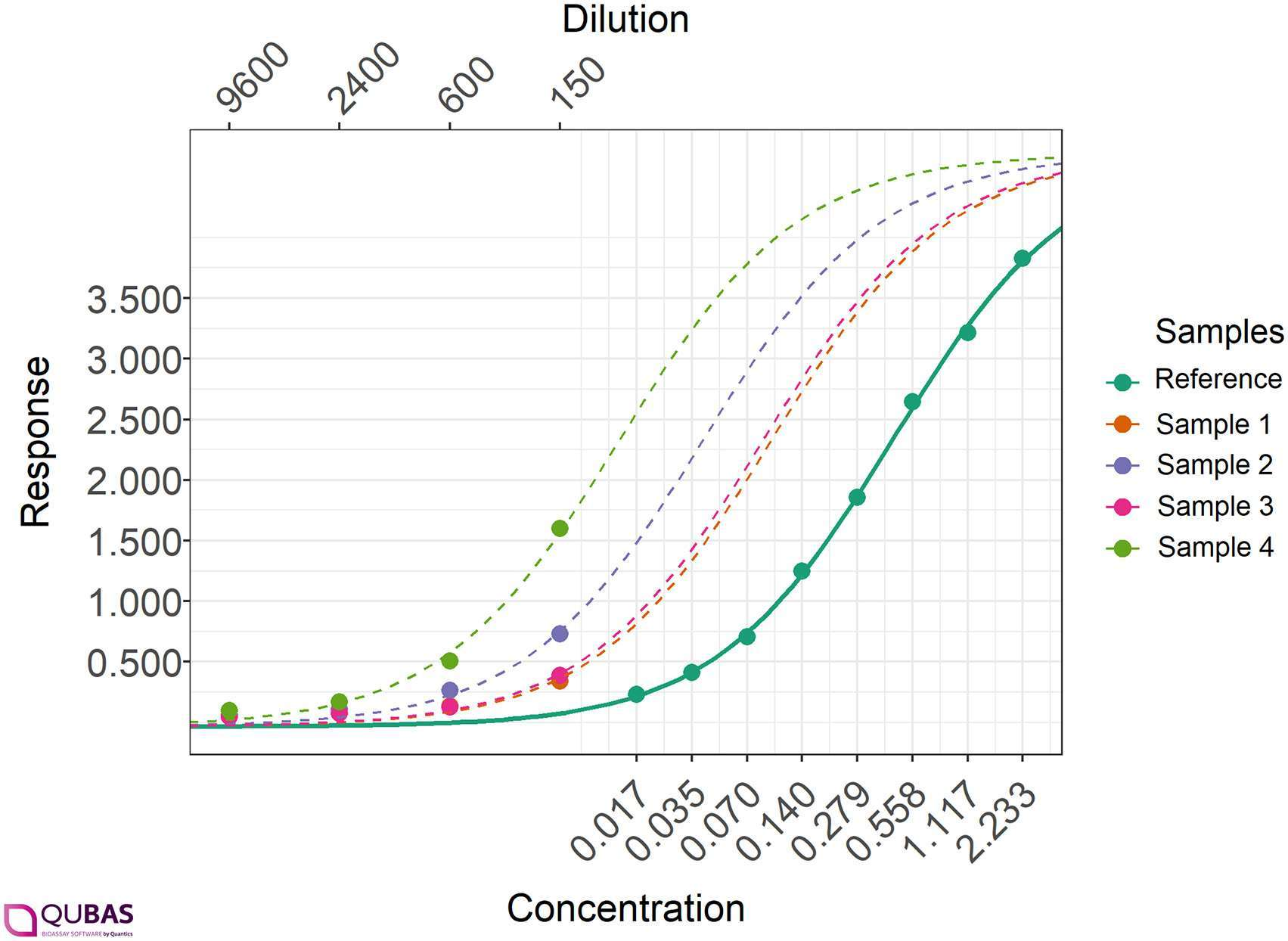Fig. 2. Example real-world human serum ELISA data. Reference fit (dark green solid curve) and test sample fits (dashed) using the new method are shown.

2.3. Comparison of the approaches

The new method improves on the traditional method. It uses all the data (including responses outside the asymptotes), but responses near the asymptotes have less influence on the fit. No abrupt cut-off is needed, and the suitability criteria can be greatly simplified. Since the responses on the flatter part of the curve do not have an excessive influence on the results, the new method is also more accurate and precise.

We used both simulations and real data to compare the accuracy and precision of the traditional and new methods. We first describe the simulations and then turn to the real data.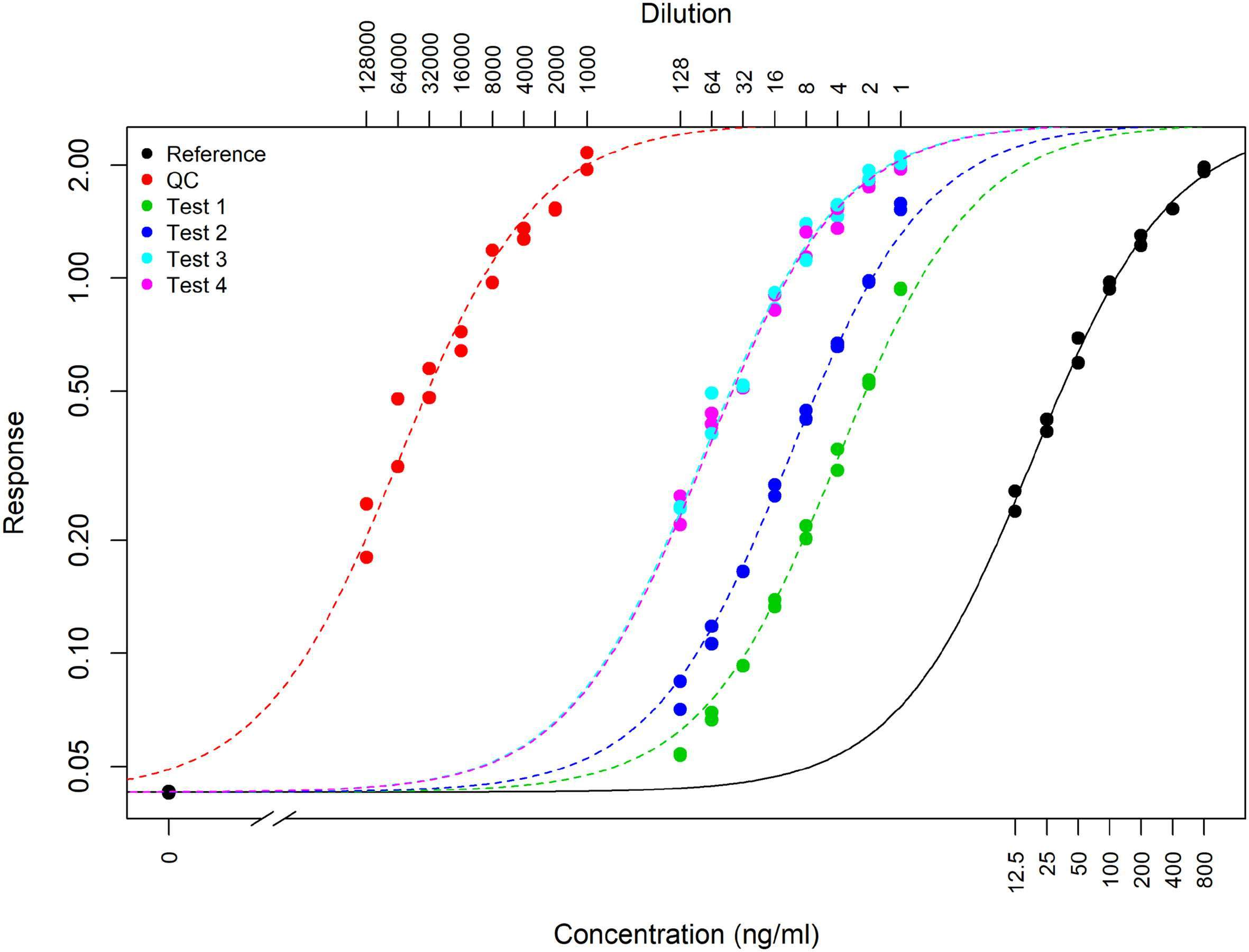Fig. 3. Example real-world PA assay. Reference fit (black solid curve) and test sample fits (dashed) using the new method are shown.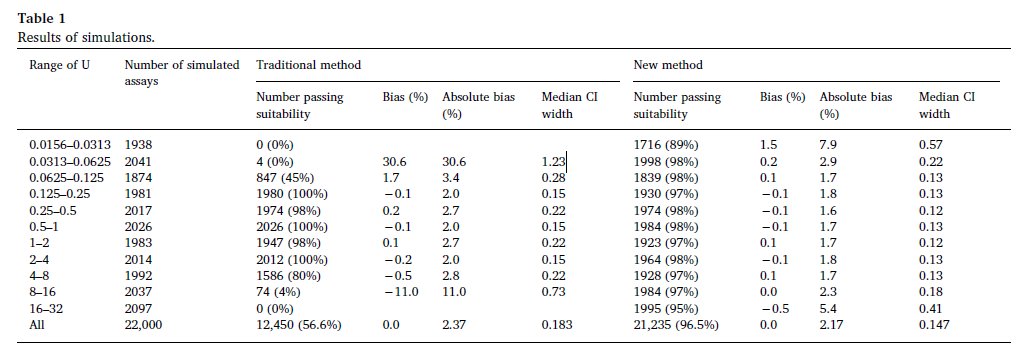2.4. Simulation study

We simulated 22,000 assays with the following parameters:

• Reference standard: 1 replicate at 8 concentrations of a 2-fold dilution series, starting at 1 unit (1, 0.5, 0.25, 0.125, 0.0625, 0.03125, 0.015625, 0.0078125)
• Test sample: 1 replicate at 4 dilutions (1:1 (i.e. neat), 1:4, 1:16 and 1:64).
• Both the reference standard and test sample follow 4PLs with parameters A = 0, B = 2 and D = 2.
• The reference EC50 is fixed at 0.1.
• Test sample concentration U uniformly distributed (on the log scale) between 0.015625 and 32.
• Responses normally distributed with standard deviation 0.02.

An example simulated assay is shown in Fig. 1.

For each simulated assay we estimate the test concentration using both the traditional and new methods. For the traditional method we exclude any test responses that are closer to one of the asymptotes than a cut-off which we set to 5% of |D − A|, and we require at least two remaining responses after this is done. For the new method we require that the test sample fit converges, and p > 0.01 for the approximate F test. For each method we also require that the precision factor must be below 4.

For each assay we calculate the estimation error, defined as ln(Û) — ln(U), the difference between the true (simulated) sample concentration and the estimate on the log scale, the accuracy, defined as the absolute value of the estimation error, and the precision, defined as the width of the 95% CI for ln(Û). The bias and the absolute bias are defined as the median values of the estimation error and the accuracy respectively.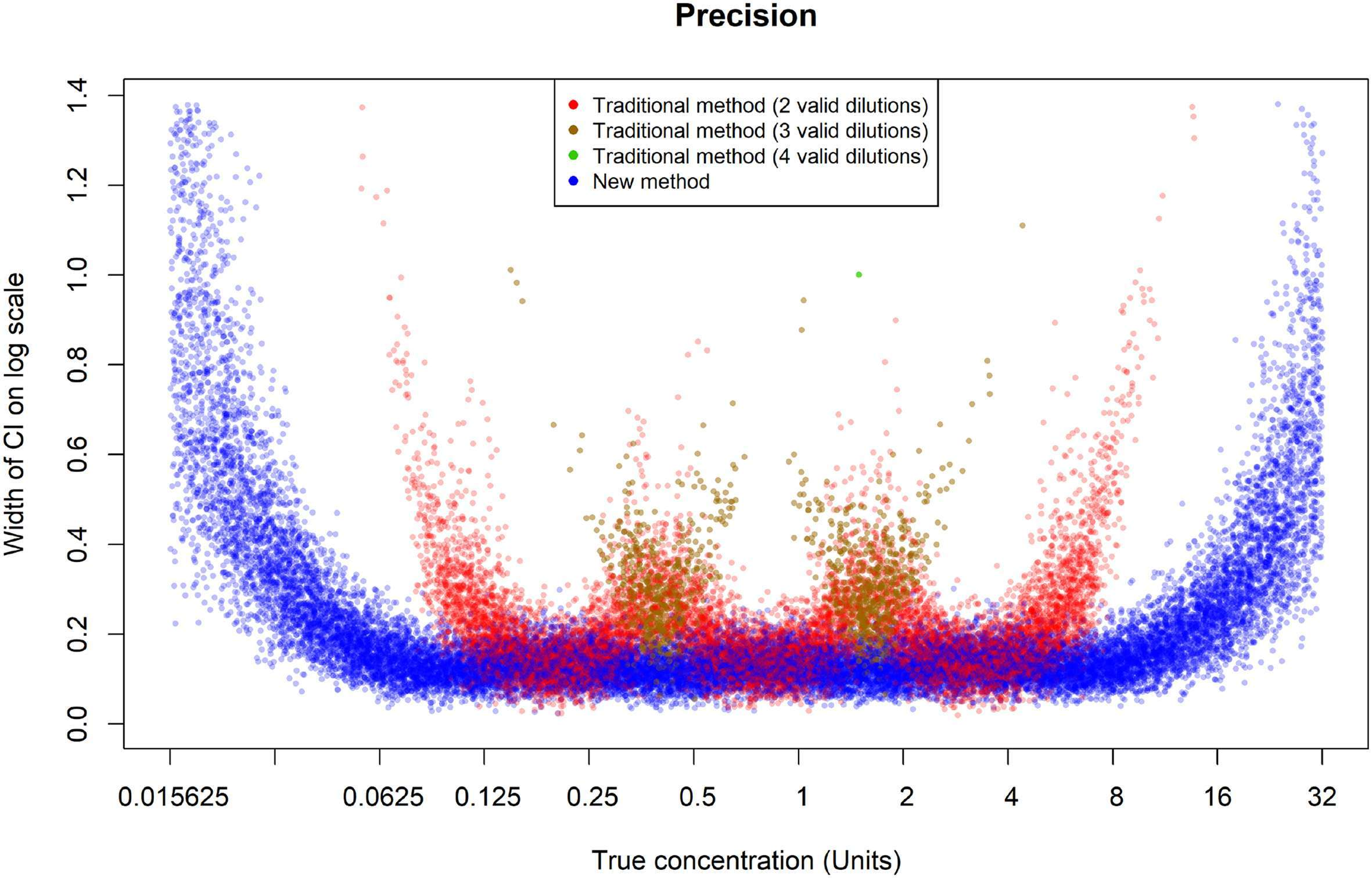Fig. 4. Precision for the two methods for the simulated assays, plotted against the true concentration.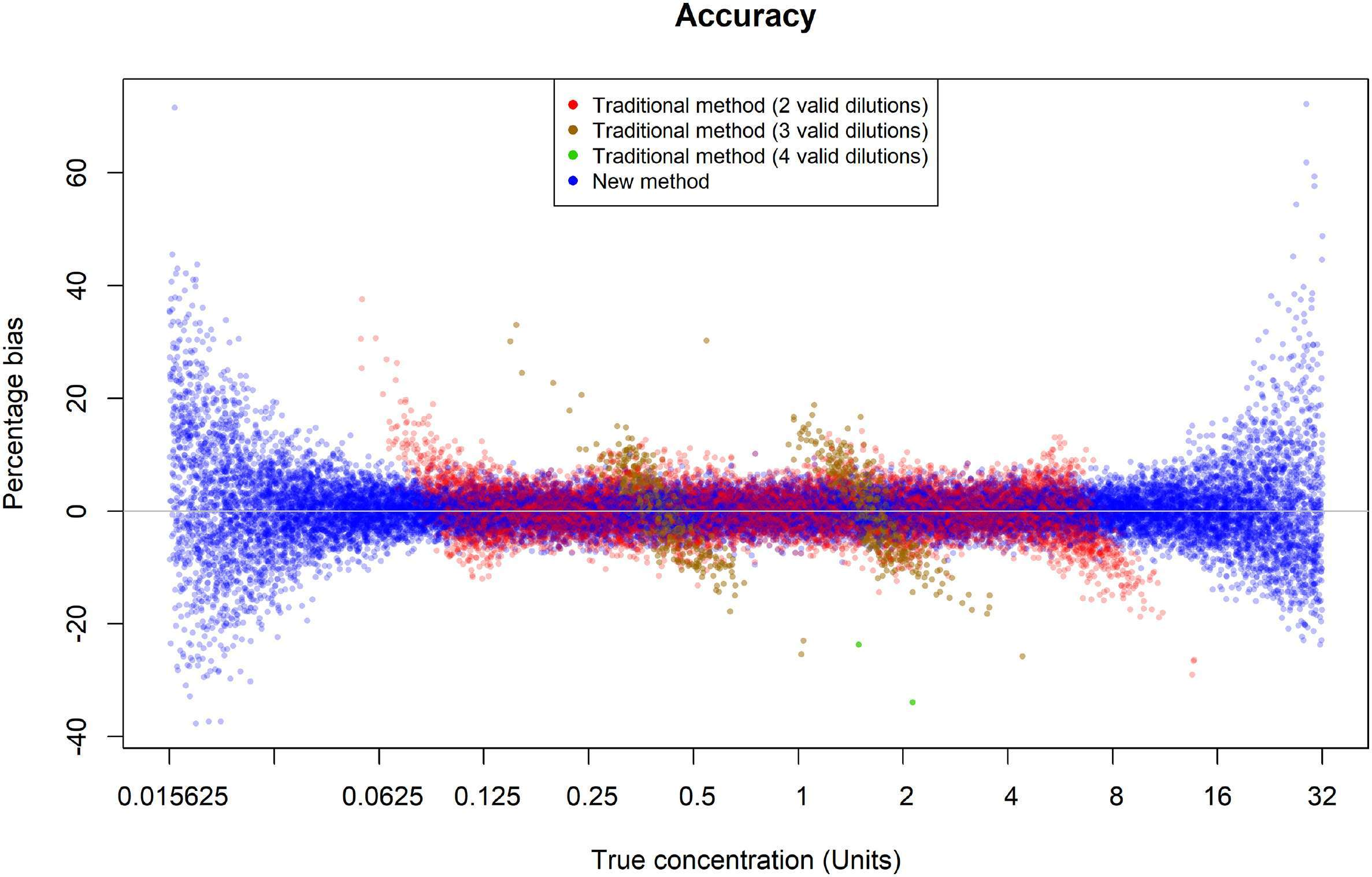Fig. 5. Estimation error for the two methods for the simulated assays, plotted against the true concentration.

2.5. Real data examples

The first real assay we analyse is a human serum ELISA.

The dataset we analysed included 98 plates, with 4 test samples per plate for a total of 392 test samples. The reference standard has 8 concentrations, and the test sample has 8 dilutions. This is somewhat unusual for ELISAs, which typically have fewer test sample dilutions. We therefore drop every second dilution and only analyse the remaining four. At each concentration of the reference standard and each test sample dilution there are two replicates; we average these before beginning the analysis.

An example of a plate from this assay is shown in Fig. 2. Note that the real assay data is somewhat different to the assay data we have simulated – the dilutions are more closely spaced together relative to the width of the reference 4PL curve, and the responses for the test samples nearly always lie on the lower part of the curve (compare Fig. 2 and Fig. 1). We may therefore expect the results of the two methods will be different for the real and simulated assays.

We also analyse a second assay, an anthrax vaccine protective antigen (PA) ELISA, which has been developed to monitor the levels of PA under different manufacturing conditions. In this study samples were taken at different times of the manufacturing process and the PA content determined. A Quality Control (QC) was also tested to verify assay functionality. The dataset includes 7 plates, each with one reference standard, one QC and four test samples. The reference standard has 8 concentrations, and the QC and test samples have 8 dilutions, all with two replicates. Again, we average the two replicates at each dilution before the analysis. For the PA assay the reference concentrations are mostly in the upper half of the 4PL curve. This is a different situation to our simulations and the human serum ELISA assay, which have full reference curves; thus we expect that the results may be somewhat different. An example of a plate from this assay is shown in Fig. 3.

We have analysed these assays using both the traditional and the new methods. We use the same suitability criteria as in the simulations. For each QC and test sample, we calculate the concentration estimates for the two methods, the precision, defined by the width of the CI for ln(Û), and whether it would have passed the suitability tests.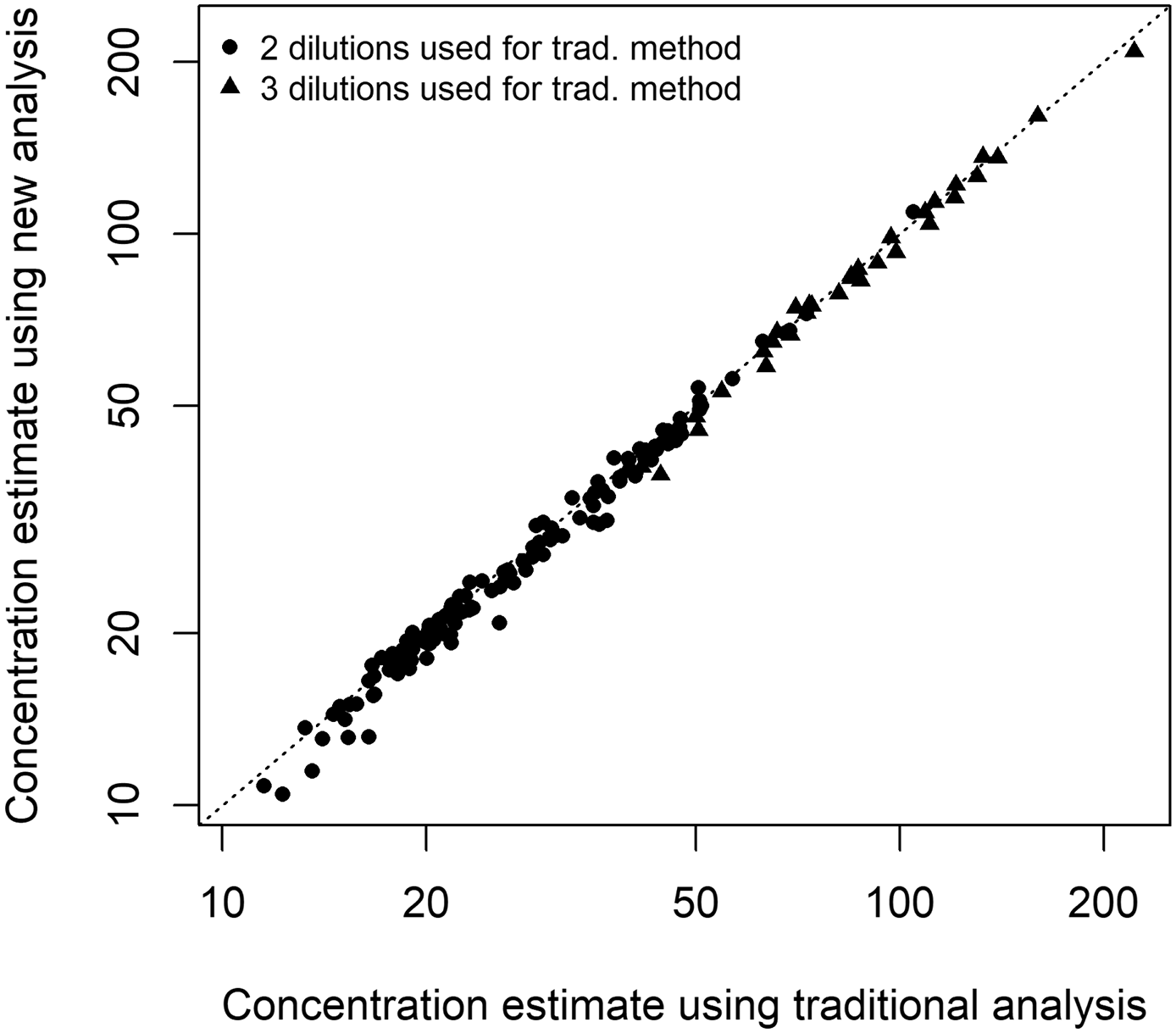Fig. 6. Results using both methods for the human serum ELISA using real-world data. Only samples passing suitability for both methods are shown.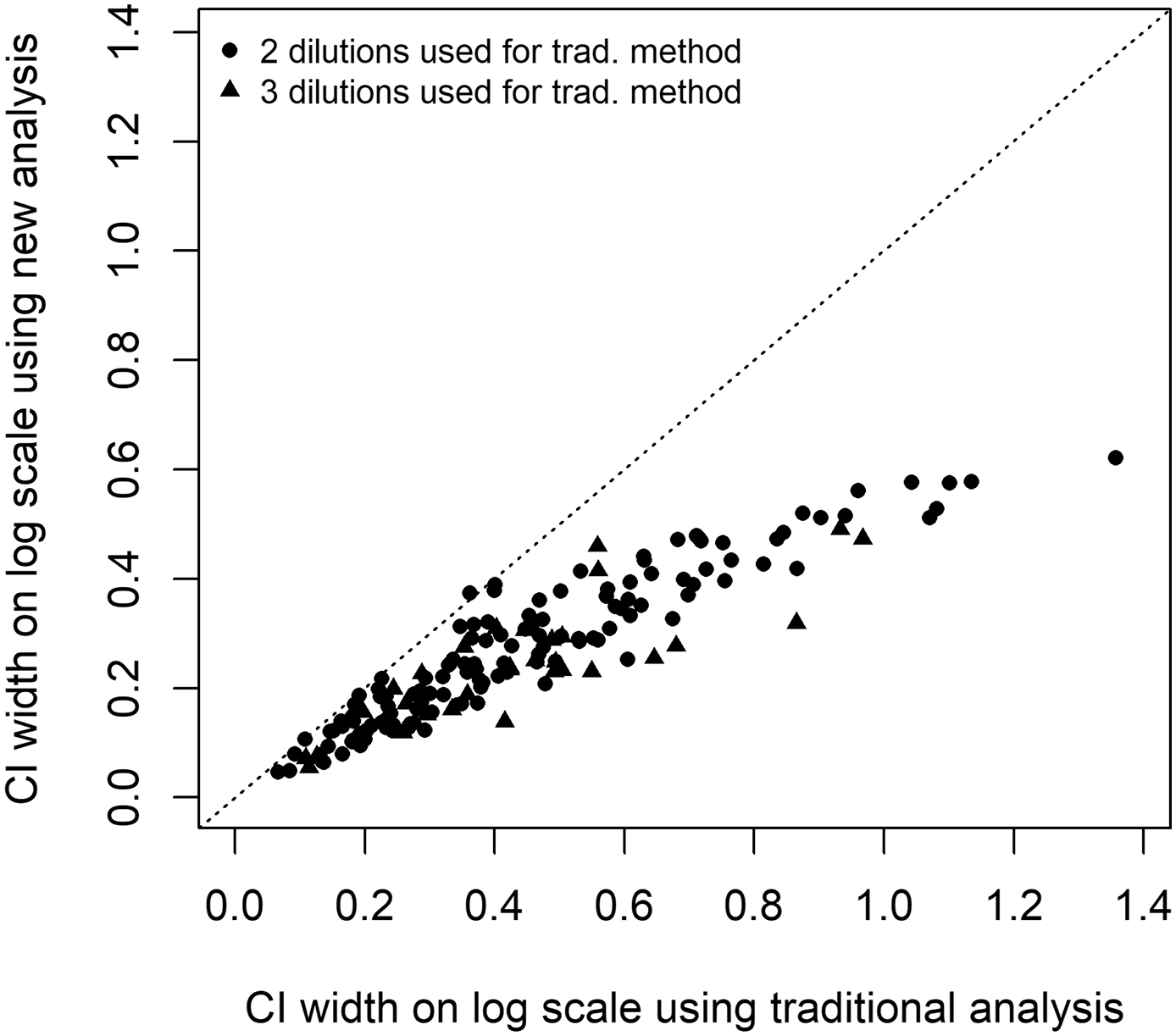Fig. 7. Precisions using both methods for the human serum ELISA using real world data. Only samples passing suitability for both methods are shown.

3. Results

3.1. Simulations

The results of the simulations are summarised in Table 1, with the results broken down by true (simulated) concentration.

Overall, the proportion of simulated assays with a valid concentration estimate is much higher with the new method (96.5%) than with the traditional method (56.6%). The accuracy is marginally better for the new method (Absolute bias = 2.2%) than for the traditional method (Absolute bias = 2.4%).

The precision is however substantially better using the new method (median CI width = 0.147) than using the traditional method (median CI width = 0.183). This includes assays which pass suitability with the new method, but not with the traditional method, which tend to have wider CIs, so this comparison disfavours the new method. Restricting the comparison to only assays which pass with both methods, the advantage of the new method is even greater: the median CI width is 0.125 compared to 0.183 for the traditional method.

The increase in the proportion of assays with a valid estimate means the need for retests is greatly reduced, and the throughput of the assay is increased by 71%. Furthermore, the improvement in precision could be traded for a decrease in the number of dilutions and/or replicates, which would increase the throughput further.

The better performance of the new method is particularly apparent in the lowest and highest bins in Table 1. Here the pass rate for the traditional method is extremely low (< 1%) because in most cases all the responses are very near the bottom asymptote (for low U) or the top asymptote (for high U). However the new method is still able to obtain a result in most cases, albeit with reduced accuracy and precision.

This is also illustrated in Fig. 4, which shows the precision achieved by each method. Each simulated sample is represented by up to two points: a red, brown or green one for the traditional method and a blue one for the new method. (If the sample failed suitability, no point is shown for that method.)

Note that there are very few results using the traditional method for true concentrations lower than about 0.1 or higher than about 10. On the other hand the new method still gives results at low and high concentrations. These are typically less precise, but may still meet precision specifications (if these have been set). Furthermore in some contexts having a result, although imprecise, is much more useful than no result at all.

Another advantage of the new method is illustrated in Fig. 5. This shows the estimation error (percentage difference between estimated and true concentration) of each method, plotted against the true (simulated, so known) concentration. The new method is, on average, unbiased; there are equal numbers of blue points above and below zero. However, the traditional method is biased at certain true concentrations. For example, for true concentrations around 0.08 it tends to give concentration estimates above the true concentration, whereas for true concentrations around 0.5 it tends to give estimates that are too low.

This bias is not very large (around 10%) but it is surprising that it is there at all. The simulated responses lie on a 4PL and are unbiased (equally likely to be above and below the expected value), and the interpolation method also assumes they lie on a 4PL so one might expect that the results will be correct on average.

The bias is in fact indirectly due to exclusion of low and high responses. The “spikes” occur at concentrations where one of the test responses is very near the exclusion limits (recall we have set these at 5% of the distance between the asymptotes). For example, at a true concentration of 0.5, the mean response for the 1:1 test dilution is 1.92. The cut-off for exclusion is 1.90 on average, so for most test samples this dilution will be excluded and the analysis will only use the 1:4 and 1:16 dilutions (the 1:64 dilution will always be too near the lower asymptote to be included). However sometimes the 1:1 dilution will be lower than usual and will be included in the analysis; since this only happens when the response is below average this results in a downward bias in Û. In this case three dilutions will be used so the corresponding point in Fig. 5 will be brown, rather than red, and indeed the brown points around a true concentration of 0.5 tend to have a negative bias.

Similarly, the other “spikes” in Fig. 5 occur when one of the dilutions is just at the edge of being excluded. There is no such issue for the new analysis method since there is no sharp cut-off where data is excluded. Instead, the influence of each data point is gradually reduced as it comes closer to one of the asymptotes.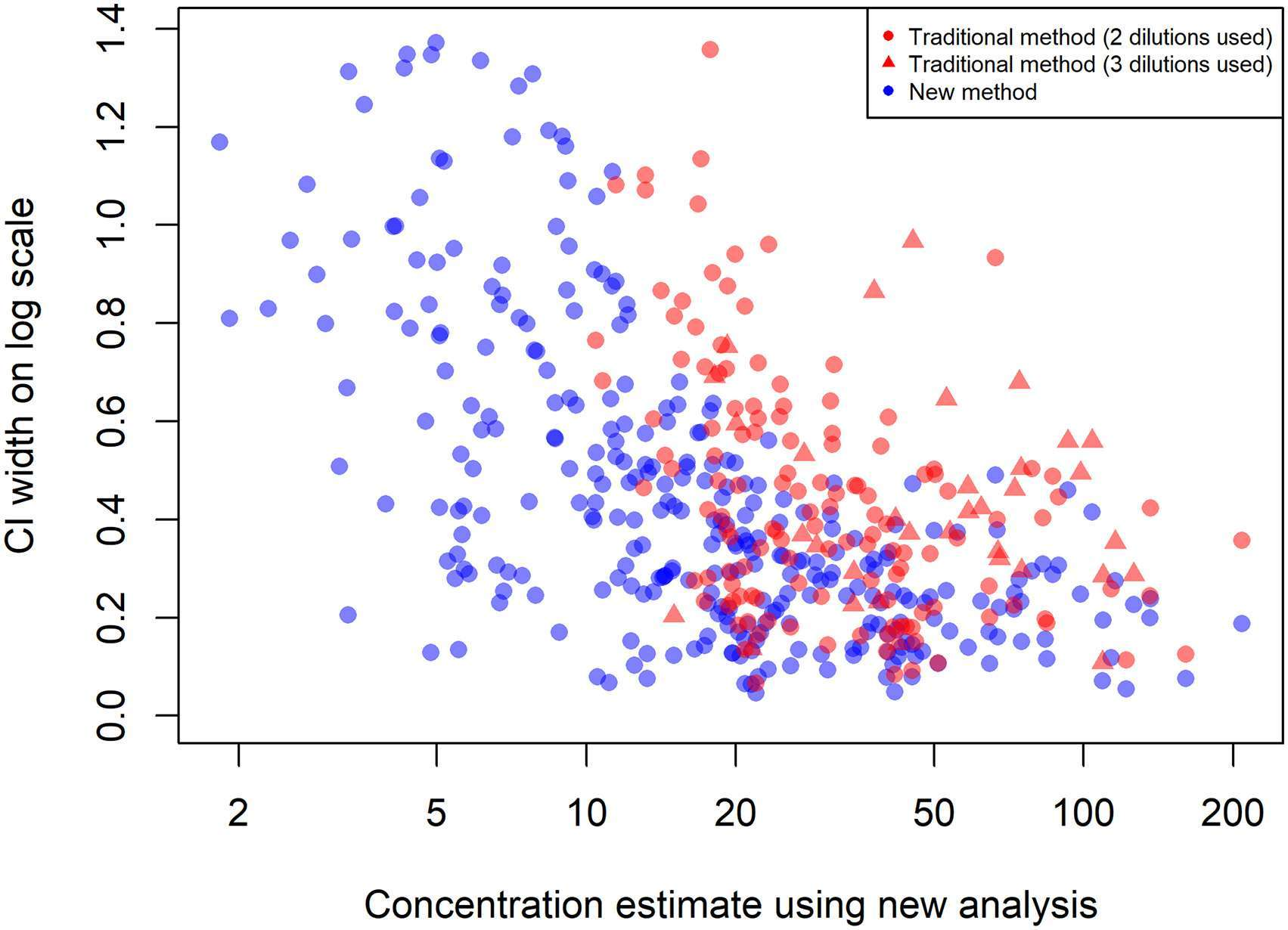Fig. 8. Precision for the human serum ELISA using the traditional (red) and new (blue) methods, plotted against the concentration estimate using the new method.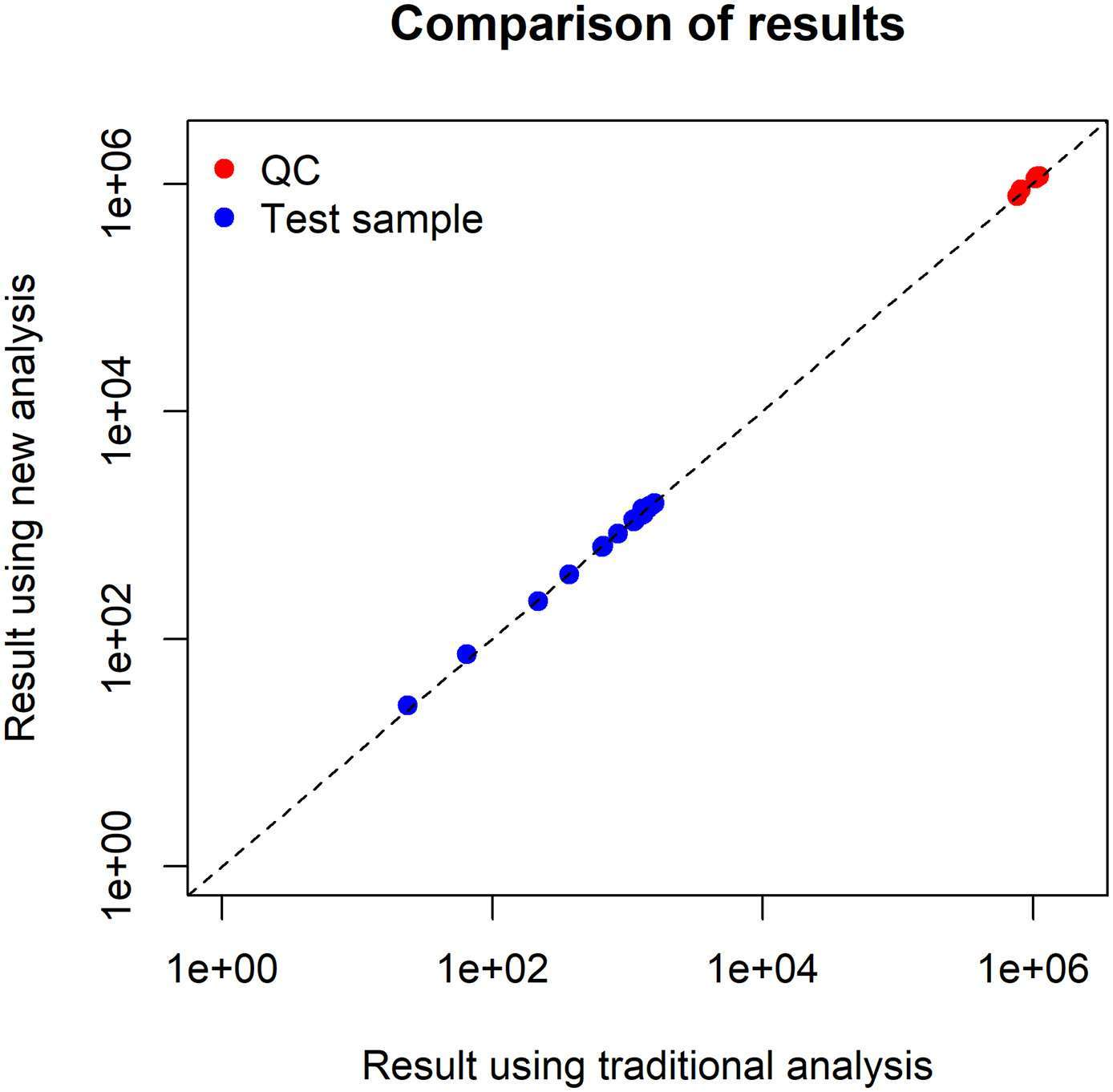Fig. 9. Results using both methods for the PA assay. Only samples passing suitability for both methods are shown.

3.2. Real-world data

Real world data incorporates many different practical aspects which contribute to variability i.e. different days, operators, equipment and reagents. The results for the human serum ELISA using the traditional and new methods are compared in Fig. 6. It is clear there is usually very good agreement between the methods. The median difference between the estimates using the two methods is 2.6%, indicating there is only a very small overall difference between the methods. Furthermore, the median absolute difference is only 3.5%, so the difference for individual samples is also small.

We compare the precisions for the two methods in Fig. 7. The new method is clearly more precise: the confidence interval is narrower for 163 of the 164 samples which pass sample suitability using both methods, and the median decrease in the CI width is 41%.

Apart from the improvement in precision a further advantage of the new method is shown in Fig. 8 below. This compares the precisions for the new method (in blue) and the traditional method (red). As in Fig. 5, these are plotted against the concentration estimate (using the new method); samples which pass suitability for both methods will have two points above each other, and those that pass only using one of the methods will have only a single point of the appropriate colour.

We see that for moderate and high sample concentrations both methods give results that pass the suitability tests. The CIs for the new method are typically narrower than for the traditional method, consistent with the improved precision seen in Fig. 7. However at low concentrations only the new method gives results; the traditional method will typically have too few valid responses at such concentrations. This is consistent with the behaviour seen in simulations (see Fig. 4).

There is also an improvement in the proportion of samples passing suitability. For the traditional method, only 170 of 392 samples (43%) pass the suitability tests; for the new method this almost doubles to 311 samples (79%). Again, this is mainly due to the fact that the new method gives results at low concentrations where the traditional method usually fails suitability.

For the PA assay, the results using the two methods are compared in Fig. 9. Again, there is very good agreement between the methods, over a wide range of true concentrations. The median difference between the estimates using the two methods is only 1.2%, and the median absolute difference is 2.0%, so the difference between the methods is small.

We compare the precisions for the two methods in Fig. 10. The new method is clearly more precise: the confidence interval is narrower for all 25 samples which pass sample suitability with both methods, and the median decrease in the CI width is 39%.

In this case there is little difference in the sample suitability pass rates. For the traditional method, 29 of the 35 samples (83%) pass sample suitability, whereas for the new method 27 (77%) pass. The lack of improvement for the new method may be due to the fact that there are few samples with very low concentrations where the traditional method would be more likely to fail suitability.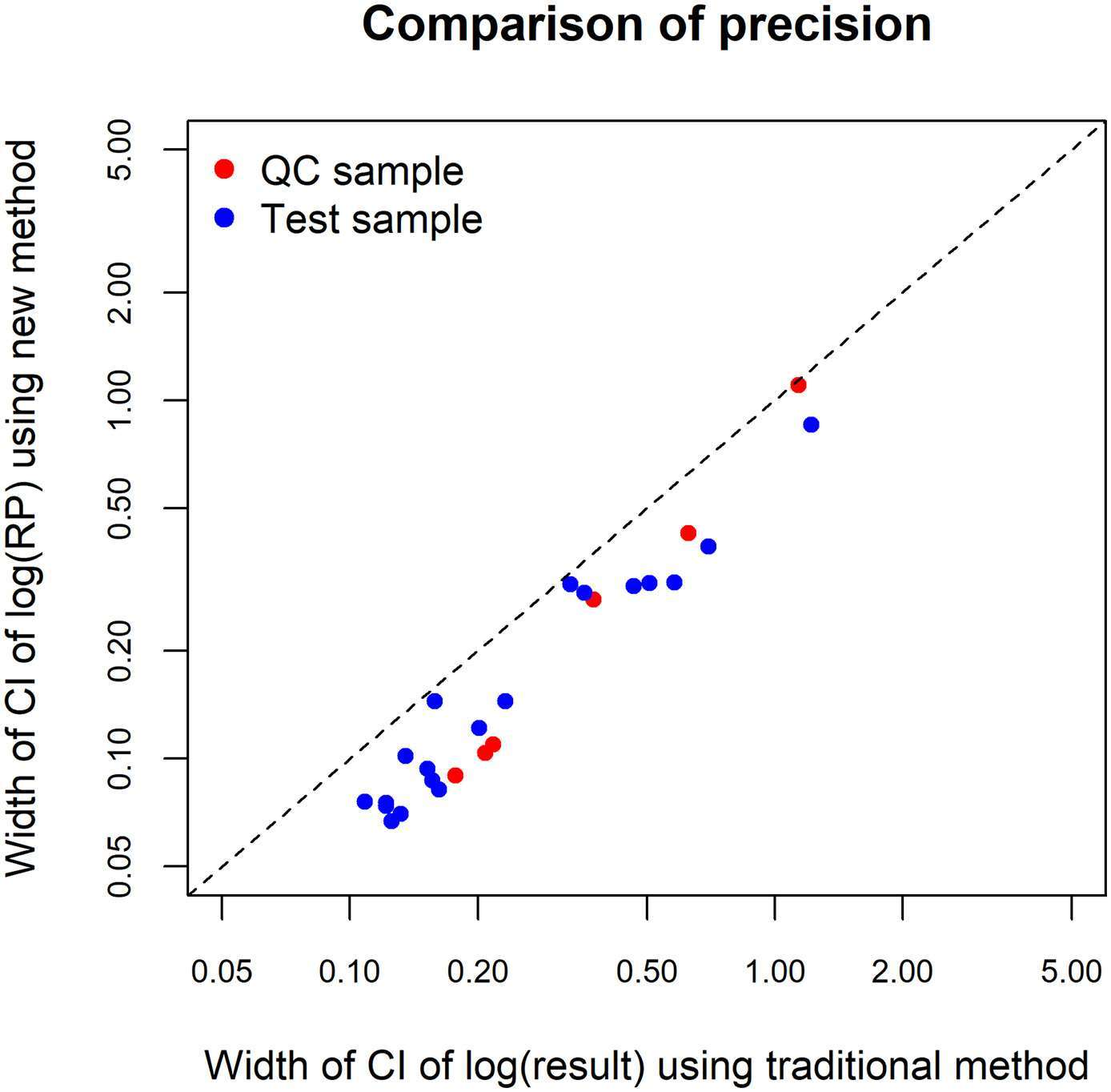Fig. 10. Precision using both methods for the PA assay. Only samples passing suitability for both methods are shown.

4. Conclusions

In this paper we have introduced a new method for analysis of ELISAs that improves on the traditional method. In particular, it uses all of the data without the need for an abrupt cut-off excluding responses near the asymptotes, and it does not give undue weight to responses on the flatter parts of the dose-response curve.

The new method is more accurate and precise in principle than the traditional method. Our simulation and real-world results show that this is also true in practice, at least for the particular cases we have considered. In particular, the new method works much better than the traditional method for very low-concentration or high-concentration samples, for which the traditional method is often unable to give a result. We have covered a wide range of scenarios across both simulations and the real-world assays, so it is plausible that the new method will be an improvement in general. One limitation of this work is that we have only considered the 4PL model; while it would be expected that the new method will also work better for other models such as the linear model and five-parameter logistic, more work would be needed to assess exactly how large the improvement would be.

A further advantage of our method is that it can be extended to more sophisticated models e.g. those incorporating mode complicated variance structures, row and column effects, etc. (Lansky, 2012; Rocke and Lorenzato, 1995; Higgins et al., 1998); it would be very difficult to account for such behaviour with the traditional method.

The increased precision possible with the new method could be traded off against a reduction in the number of dilutions and/or replicates to increase the throughput of an assay. For the human serum ELISA, we have shown that the median decrease in the width of the 95% CI for the concentration (on the log scale) is 41%; if the number of replicates or dilutions was halved, the throughput of the assay would be doubled and there would still be a 17% decrease in the width of the CI compared to using the traditional method with the original design. In addition, there is a further gain in the throughput due to the higher pass rate for suitability tests, which avoids the need for retests. Finally, since CIs are rarely calculated for the traditional method, the process of changing methods may reveal that the assay is more precise than is necessary for its intended purpose; if so there will be further room for increasing throughput by decreasing the number of dilutions and/or replicates.

Given the improvement in performance of the new method there seems little reason not to change to the new method. Migration from the traditional to the new analysis methods can be managed in a similar way to e.g. a bridging study.

The precision referred to in this publication is the precision around one measurement of a test sample (U). A comparison of intra- and interassay precision for the new analysis method and the traditional method would have to be done with real-word data to establish whether this aspect of assay performance is also improved.